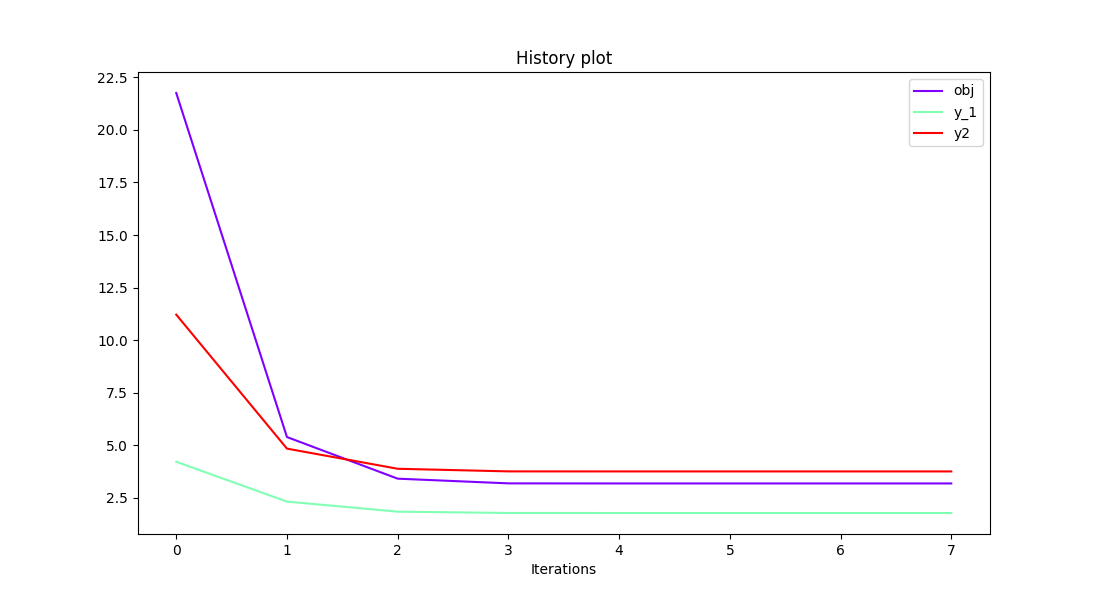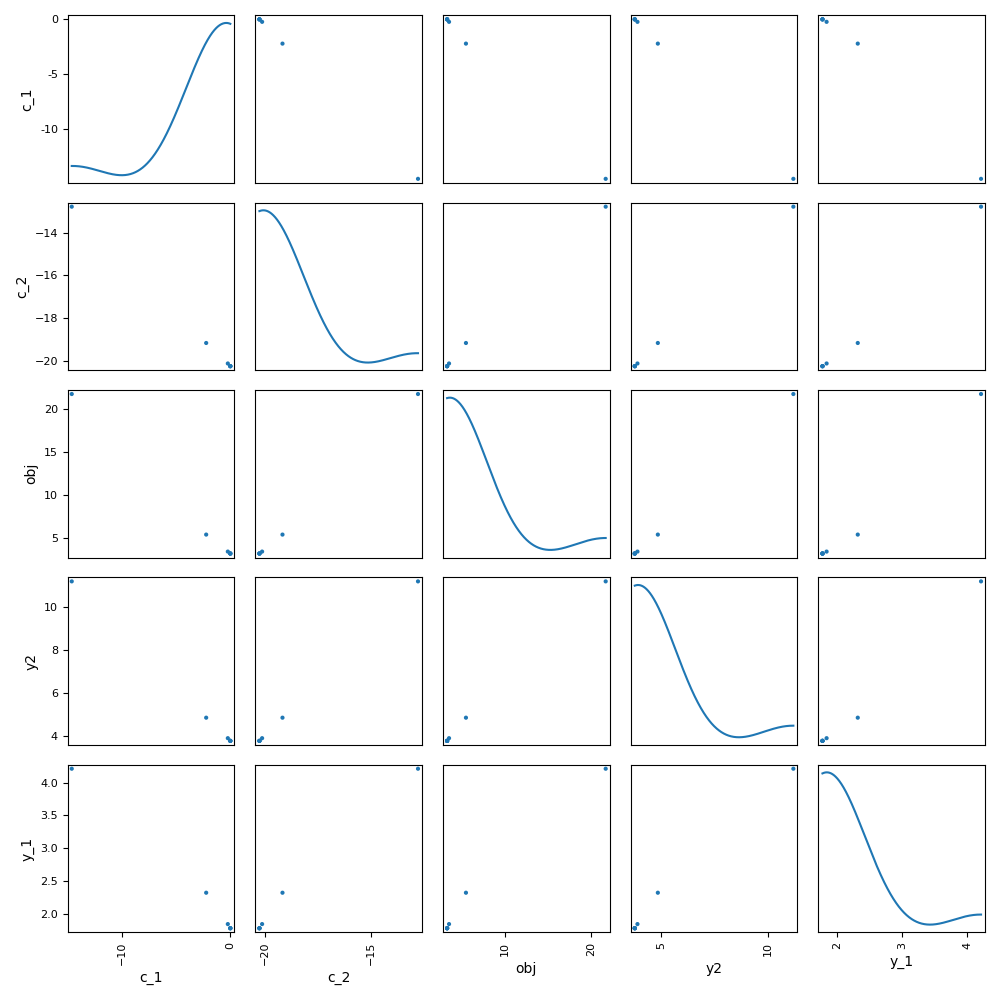# Store observables¶

## Introduction¶

In this example, we will learn how to store the history of state variables using the add_observable() method. This is useful in situations where we wish to access, post-process, or save the values of discipline outputs that are not design variables, constraints or objective functions.

## The Sellar problem¶

We will consider in this example the Sellar problem:

\begin{split}\begin{aligned} \text{minimize the objective function }&obj=x_{local}^2 + x_{shared,2} +y_1^2+e^{-y_2} \\ \text{with respect to the design variables }&x_{shared},\,x_{local} \\ \text{subject to the general constraints } & c_1 \leq 0\\ & c_2 \leq 0\\ \text{subject to the bound constraints } & -10 \leq x_{shared,1} \leq 10\\ & 0 \leq x_{shared,2} \leq 10\\ & 0 \leq x_{local} \leq 10. \end{aligned}\end{split}

where the coupling variables are

$\text{Discipline 1: } y_1 = \sqrt{x_{shared,1}^2 + x_{shared,2} + x_{local} - 0.2\,y_2},$

and

$\text{Discipline 2: }y_2 = |y_1| + x_{shared,1} + x_{shared,2}.$

and where the general constraints are

\begin{align}\begin{aligned}c_1 = 3.16 - y_1^2\\c_2 = y_2 - 24.\end{aligned}\end{align}

## Imports¶

All the imports needed for the tutorials are performed here.

from __future__ import annotations

from gemseo.algos.design_space import DesignSpace
from gemseo.api import configure_logger
from gemseo.api import create_discipline
from gemseo.api import create_scenario
from numpy import array
from numpy import ones

configure_logger()

<RootLogger root (INFO)>


## Create the problem disciplines¶

In this section, we use the available classes Sellar1, Sellar2 and SellarSystem to define the disciplines of the problem. The create_discipline() API function allows us to carry out this task easily, as well as store the instances in a list to be used later on.

disciplines = create_discipline(["Sellar1", "Sellar2", "SellarSystem"])


## Create and execute the scenario¶

### Create the design space¶

In this section, we define the design space which will be used for the creation of the MDOScenario.

design_space = DesignSpace()
"x_shared", 2, l_b=(-10, 0.0), u_b=(10.0, 10.0), value=array([4.0, 3.0])
)


### Create the scenario¶

In this section, we build the MDO scenario which links the disciplines with the formulation, the design space and the objective function.

scenario = create_scenario(
disciplines, formulation="MDF", objective_name="obj", design_space=design_space
)


Then, we have to set the design constraints

scenario.add_constraint("c_1", "ineq")


Only the design variables, objective function and constraints are stored by default. In order to be able to recover the data from the state variables, y1 and y2, we have to add them as observables. All we have to do is enter the variable name as a string to the add_observable() method. If more than one output name is provided (as a list of strings), the observable function returns a concatenated array of the output values.

scenario.add_observable("y_1")


It is also possible to add the observable with a custom name, using the option observable_name. Let us store the variable y_2 as y2.

scenario.add_observable("y_2", observable_name="y2")


### Execute the scenario¶

Then, we execute the MDO scenario with the inputs of the MDO scenario as a dictionary. In this example, the gradient-based SLSQP optimizer is selected, with 10 iterations at maximum:

scenario.execute(input_data={"max_iter": 10, "algo": "SLSQP"})

    INFO - 16:58:01:
INFO - 16:58:01: *** Start MDOScenario execution ***
INFO - 16:58:01: MDOScenario
INFO - 16:58:01:    Disciplines: Sellar1 Sellar2 SellarSystem
INFO - 16:58:01:    MDO formulation: MDF
INFO - 16:58:01: Optimization problem:
INFO - 16:58:01:    minimize obj(x_local, x_shared)
INFO - 16:58:01:    with respect to x_local, x_shared
INFO - 16:58:01:    subject to constraints:
INFO - 16:58:01:       c_1(x_local, x_shared) <= 0.0
INFO - 16:58:01:       c_2(x_local, x_shared) <= 0.0
INFO - 16:58:01:    over the design space:
INFO - 16:58:01:    +-------------+-------------+-------+-------------+-------+
INFO - 16:58:01:    | name        | lower_bound | value | upper_bound | type  |
INFO - 16:58:01:    +-------------+-------------+-------+-------------+-------+
INFO - 16:58:01:    | x_local     |      0      |   1   |      10     | float |
INFO - 16:58:01:    | x_shared |     -10     |   4   |      10     | float |
INFO - 16:58:01:    | x_shared |      0      |   3   |      10     | float |
INFO - 16:58:01:    +-------------+-------------+-------+-------------+-------+
INFO - 16:58:01: Solving optimization problem with algorithm SLSQP:
INFO - 16:58:01: ...   0%|          | 0/10 [00:00<?, ?it]
INFO - 16:58:01: ...  10%|█         | 1/10 [00:00<00:00, 35.68 it/sec, obj=21.8+j]
slsqp(m, meq, x, xl, xu, fx, c, g, a, acc, majiter, mode, w, jw,
INFO - 16:58:01: ...  20%|██        | 2/10 [00:00<00:00, 30.99 it/sec, obj=5.39+j]
INFO - 16:58:01: ...  30%|███       | 3/10 [00:00<00:00, 30.53 it/sec, obj=3.41+j]
INFO - 16:58:01: ...  40%|████      | 4/10 [00:00<00:00, 30.46 it/sec, obj=3.19+j]
INFO - 16:58:01: ...  50%|█████     | 5/10 [00:00<00:00, 30.43 it/sec, obj=3.18+j]
INFO - 16:58:01: ...  60%|██████    | 6/10 [00:00<00:00, 30.35 it/sec, obj=3.18+j]
INFO - 16:58:01: ...  70%|███████   | 7/10 [00:00<00:00, 29.93 it/sec, obj=3.18+j]
INFO - 16:58:01: ...  80%|████████  | 8/10 [00:00<00:00, 32.85 it/sec, obj=3.18+j]
INFO - 16:58:01: Optimization result:
INFO - 16:58:01:    Optimizer info:
INFO - 16:58:01:       Status: None
INFO - 16:58:01:       Message: Successive iterates of the objective function are closer than ftol_rel or ftol_abs. GEMSEO Stopped the driver
INFO - 16:58:01:       Number of calls to the objective function by the optimizer: 9
INFO - 16:58:01:    Solution:
INFO - 16:58:01:       The solution is feasible.
INFO - 16:58:01:       Objective: (3.1833939452393087+0j)
INFO - 16:58:01:       Standardized constraints:
INFO - 16:58:01:          c_1 = (6.486713388653698e-09+0j)
INFO - 16:58:01:          c_2 = (-20.244722236724627+0j)
INFO - 16:58:01:       Design space:
INFO - 16:58:01:       +-------------+-------------+-------------------+-------------+-------+
INFO - 16:58:01:       | name        | lower_bound |       value       | upper_bound | type  |
INFO - 16:58:01:       +-------------+-------------+-------------------+-------------+-------+
INFO - 16:58:01:       | x_local     |      0      |         0         |      10     | float |
INFO - 16:58:01:       | x_shared |     -10     | 1.977638881636786 |      10     | float |
INFO - 16:58:01:       | x_shared |      0      |         0         |      10     | float |
INFO - 16:58:01:       +-------------+-------------+-------------------+-------------+-------+
INFO - 16:58:01: *** End MDOScenario execution (time: 0:00:00.255756) ***

{'max_iter': 10, 'algo': 'SLSQP'}


## Access the observable variables¶

### Retrieve observables from a dataset¶

In order to create a dataset, we use the corresponding OptimizationProblem:

opt_problem = scenario.formulation.opt_problem


We can easily build a dataset from this OptimizationProblem: either by separating the design parameters from the functions (default option):

dataset = opt_problem.export_to_dataset("sellar_problem")
print(dataset)

sellar_problem
Number of samples: 8
Number of variables: 7
Variables names and sizes by group:
design_parameters: x_local (1), x_shared (2)
functions: c_1 (1), c_2 (1), obj (1), y2 (1), y_1 (1)
Number of dimensions (total = 8) by group:
design_parameters: 3
functions: 5


or by considering all features as default parameters:

dataset = opt_problem.export_to_dataset("sellar_problem", categorize=False)
print(dataset)

sellar_problem
Number of samples: 8
Number of variables: 7
Variables names and sizes by group:
parameters: c_1 (1), c_2 (1), obj (1), x_local (1), x_shared (2), y2 (1), y_1 (1)
Number of dimensions (total = 8) by group:
parameters: 8


or by using an input-output naming rather than an optimization naming:

dataset = opt_problem.export_to_dataset("sellar_problem", opt_naming=False)
print(dataset)

sellar_problem
Number of samples: 8
Number of variables: 7
Variables names and sizes by group:
inputs: x_local (1), x_shared (2)
outputs: c_1 (1), c_2 (1), obj (1), y2 (1), y_1 (1)
Number of dimensions (total = 8) by group:
inputs: 3
outputs: 5


#### Access observables by name¶

We can get the observable data by name, either as a dictionary indexed by the observable names (default option):

print(dataset.get_data_by_names(["y_1", "y2"]))

{'y_1': array([[4.21393092],
[2.32004903],
[1.84120131],
[1.77871073],
[1.77763921],
[1.77763888],
[1.77763888],
[1.77763888]]), 'y2': array([[11.21393092],
[ 4.84009806],
[ 3.88161141],
[ 3.75742147],
[ 3.75527841],
[ 3.75527777],
[ 3.75527776],
[ 3.75527777]])}


or as an array:

print(dataset.get_data_by_names(["y_1", "y2"], False))

[[ 4.21393092 11.21393092]
[ 2.32004903  4.84009806]
[ 1.84120131  3.88161141]
[ 1.77871073  3.75742147]
[ 1.77763921  3.75527841]
[ 1.77763888  3.75527777]
[ 1.77763888  3.75527776]
[ 1.77763888  3.75527777]]


### Use the observables in a post-processing method¶

Finally, we can generate plots with the observable variables. Have a look at the Basic History plot and the Scatter Plot Matrix:

scenario.post_process(
"BasicHistory",
variable_names=["obj", "y_1", "y2"],
save=False,
show=True,
)
scenario.post_process(
"ScatterPlotMatrix",
variable_names=["obj", "c_1", "c_2", "y2", "y_1"],
save=False,
show=True,
)

••/home/docs/checkouts/readthedocs.org/user_builds/gemseo/envs/4.3.0.post0/lib/python3.9/site-packages/gemseo/algos/database.py:1369: VisibleDeprecationWarning: Creating an ndarray from ragged nested sequences (which is a list-or-tuple of lists-or-tuples-or ndarrays with different lengths or shapes) is deprecated. If you meant to do this, you must specify 'dtype=object' when creating the ndarray.
f_history = array(f_flat_values).real

<gemseo.post.scatter_mat.ScatterPlotMatrix object at 0x7fbc39c5a880>


Total running time of the script: ( 0 minutes 1.476 seconds)

Gallery generated by Sphinx-Gallery